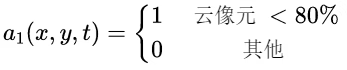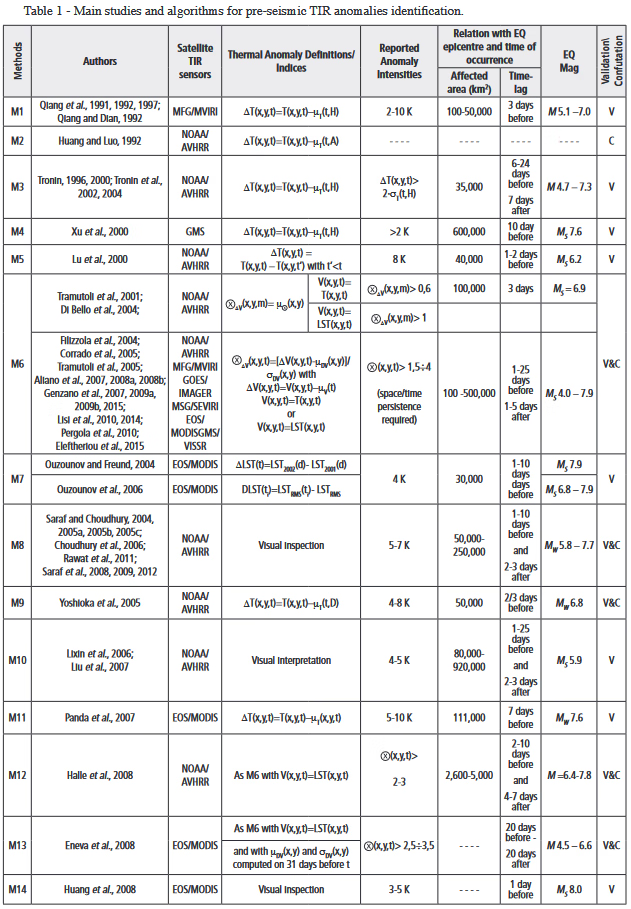## 一、多参数热异常提取

• 基于地表温度的热异常提取
• 基于长波辐射的热异常提取
• 基于NCEP(National Centers for Environmental Prediction，国家环境预报中心)的热异常提取
• 其他参量：亮度温度、总电子含量、大气温度和潜热通量等

## 三、RST(Robust Satellite Techniques，卫星数据稳健分析技术)

### 3.1 RST算法

RST算法由RAT算法演化而来，可广泛用于多种遥感数据源，该方法需要构建稳健背景场，运用遥感数据值类高斯分布特征，提取相对于背景场的偏离程度，可以有效提取热异常，在多种情况下的异常提取均可应用，如云检测、火山、森林火灾监测等方面。RST 是一种基于多时相数据分析方法，它考虑的是时空域上相对于平静状态下的异常，长时序的卫星数据用来构建信号的正常背景，以此来计算局部偏差指数，能够尽可能地去除地形、气象等因素影响。RST 算法流程首先将输入的遥感数据构建差值数据集，差值数据集包括空间域和时间域差值，进一步构建单像元背景场，根据数据特征选取热异常提取阈值，根据热异常判断方法判定热异常是否是有效热异常。 Tramutoli等（2005）改进RST算法并将其应用于地震前热红外监测，核心参数也由单一的亮度温度演化出不同类型。该方法将地震引起的热红外异常作为信号，其它因素引起的异常作为噪声，引入了地震热异常信噪比的概念（Eleftheriou et al，2016）。该算法以多年同期的遥感卫星数据为基础，提取出相对于背景场异常值，公式如下：

$R E T I R A=\frac{\Delta T(x, y, t)-\mu_{\Delta T}(x, y)}{\sigma_{\Delta T}(x, y)}$

$\Delta T(x, y, t)=T(x, y, t)-T(t)$

RETIRA(Robust Estimator of TIR Anomalies，热异常稳健估计值)为热异常稳健估计值，ΔT(x,y,t)是相对于区域均值的差值，μΔT(x,y)为ΔT(x,y,t)多年相同位置、相同时刻的平均值，σΔT(x,y)为对应的标准差，T(t)为t时刻影像均值。由于在构建背景场所用到的数据中有大量的干扰噪声，需进一步去除：

$A(x, y, t)=a_1(x, y, t) \cdot a_2(x, y, t)$a2(x, y, t)用于剔除空间异常值，对于V(x, y, t)时间序列中，与均值之差大于 k(k≥2)倍标准差的值不能用于构建背景场，应予以剔除，将a2(x, y, t)赋值为0，不断迭代直到所有数值与均值之差小于k倍中误差。

$\mu_{\Delta T}(x, y)=\frac{\sum_{\forall t \in T} \Delta T(x, y, t) \cdot A(x, y, t)}{\sum_{\forall t \in T} \Delta T(x, y, t)}$

$\sigma_{\Delta T}(x, y)=\sqrt{\frac{\sum_{\forall t \in T}\left[\Delta T(x, y, t) \cdot A(x, y, t)-\mu_{\Delta T}(x, y)\right]^2}{\sum_{\forall t \in T} A(x, y, t)}}$

### 3.3 地震热异常判断

• 满足RETIRA阈值。该阈值根据所选取数据的特性进行选择。
• 未受覆盖云影响。数据需要无云或者经过去云操作。
• 时间连续性。在时间分布上，第一次出现热异常后七日内应至少出现一次，且两次异常分布位置相同或相邻。
• 空间连续性。在空间分布上，热异常分布应当是聚集的，在检测出的异常分布上应当在经纬度 1°×1°的范围内至少覆盖 150km²。
• 其他。

## 四、其他

### 4.1 影响TIR的主要因素### 4.2 震前TIR异常提取主要研究和算法### 4.3 SSTAs(显著性热异常序列)和地震发生之间的长期相关性分析### 参考论文

孟亚飞. 基于RST算法的地震热异常相关性研究与多时相分析[D].东华理工大学,2020.DOI:10.27145/d.cnki.ghddc.2020.000290.

Tramutoli, Valerio, et al. From Visual Comparison to Robust Satellite Techniques: 30 years of thermal infrared satellite data analyses for the study of earthquakes preparation phases,https://doi.org/10.4430/bgta0149

 Tramutoli V , Filizzola C , Genzano N , et al. Robust Satellite Techniques for Detecting Preseismic Thermal Anomalies[M].